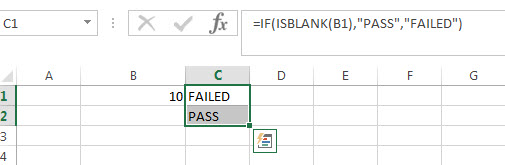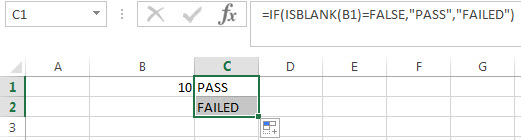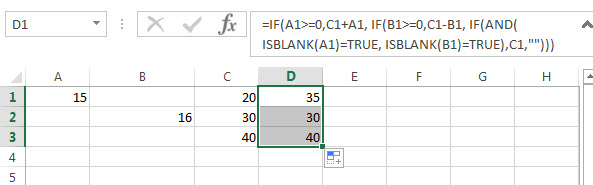# Excel IF function check if the cell is blank or not-blank

If you want to check the value in one cell if it is blank or empty cell, then you can use IF function in combination with ISBLANK function or logical operator (equal to) in excel.

## Excel IF function check if the cell is blank

Let’s see the generic IF formula with ISBLANK function and Logical operator = as follows:

```=IF(ISBLANK(B1),"PASS","FAILED")
=IF(B1="","PASS","FAILED")```

In the above two IF formulas, it will return “PASS” if Cell B1 is blank, Otherwise returns “FAILED”.## Excel IF function check if the cell is non-blank

If you want to check the value in one cell if it is not blank or not empty cell, then you can use IF function in combination with ISBLANK function or logical operator (not equal to) in excel.

let’s see the generic IF formula with ISBLANK function and Logical operator <> as follows:

```=IF(ISBLANK(B1)=FALSE,"PASS","FAILED")
=IF(B1<>"","PASS","FAILED")```

In the above two IF formulas, it will return “PASS” if Cell B1 is not blank, otherwise returns “FAILED”.Let’s write a nested if function in combining with AND function and ISBLANK function to achieve the following test criteria.

IF cell A1 is greater than or equal to 10, then add to C1.

If Cell B1 is greather than or equal to 10, then subtract from C1.

If both A1 and b1 are blank cells, then equals to C1.

Based on the above logic, we can write down the following excel nested if formula (statement):

`=IF(A1>=0,C1+A1, IF(B1>=0,C1-B1, IF(AND(ISBLANK(A1)=TRUE, ISBLANK(B1)=TRUE),C1,"")))`### Related Formulas

• Excel IF formula with operator : greater than,less than
Now we can use the above logical test with greater than operator. Let’s see the below generic if formula with greater than operator:=IF(A1>10,”excelhow.net”,”google”) …
• Excel IF function with text values
If you want to write an IF formula for text values in combining with the below two logical operators in excel, such as: “equal to” or “not equal to”…
• Excel IF Function With Numbers
If you want to check if a cell values is between two values or checking for the range of numbers or multiple values in cells, at this time, we need to use AND or OR logical function in combination with the logical operator and IF function…
• Excel IF function with Dates
If you have a list of dates and then want to compare to these dates with a specified date to check if those dates is greater than or less than that specified date. …

### Related Functions

• Excel AND function
The Excel AND function returns TRUE if all of arguments are TRUE, and it returns FALSE if any of arguments are FALSE.The syntax of the AND function is as below:= AND (condition1,[condition2],…) …
• Excel IF function
The Excel IF function perform a logical test to return one value if the condition is TRUE and return another value if the condition is FALSE….
• Excel nested if function
The nested IF function is formed by multiple if statements within one Excel if function. This excel nested if statement makes it possible for a single formula to take multiple actions…
• Excel ISBLANK function
The Excel ISBLANK function returns TRUE if the value is blank or null.The syntax of the ISBLANK function is as below:= ISBLANK (value)…

Related Posts

If Value is Greater Than A Certain Value

IF function is frequently used in Excel worksheet to return you expect “true value” or “false value” based on the logical test result. If you want to see if a value in one cell is greater than a specific value, ...

Excel IF Function With Dates

If you have a list of dates and then want to compare to these dates with a specified date to check if those dates is greater than or less than that specified date. You can use the IF function in ...

Excel IF function with text values

Normally, If you want to write an IF formula for text values in combining with the below two logical operators in excel, such as: “equal to” or “not equal to”. Excel IF function check if a cell contains text(case-insensitive) By default, ...

Excel IF Function With Numbers

Normally, If you want to write an IF formula for numbers in combining with the  logical operators in excel, such as: greater than, less than, greater than or equal to, less than or equal to, equal to, not equal to.etc. ...

Excel IF formula with NOT logical function

This post will guide you how to use excel if formula with NOT logical function in Microsoft excel. NOT Logical Function The syntax of NOT logical function is as follow: =NOT(logical_value) The NOT logical function will return the reversed logical ...

Sidebar